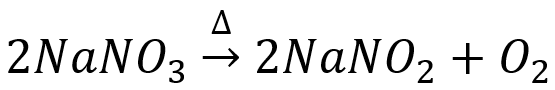# Problem: Determine the number of moles and the mass requested for each reaction in each of the following:(c) The number of moles and the mass of sodium nitrate, NaNO 3, required to produce 128 g of oxygen. (NaNO2 is the other product.)

###### FREE Expert Solution

First, let us write the balanced equation for the reaction. Our reactant is NaNO3 and our products are NaNO2 and oxygen in the form of O2.88% (173 ratings)###### Problem Details

Determine the number of moles and the mass requested for each reaction in each of the following:

(c) The number of moles and the mass of sodium nitrate, NaNO 3, required to produce 128 g of oxygen. (NaNO2 is the other product.)